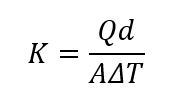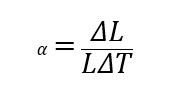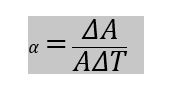# Carbon Fiber Thermal Conductivity and the Coefficient of Thermal Expansion

When designing a new product or application, engineers have multiple options for materials. Properly analyzing all material properties, while putting them into the context of the end product or application, is an extremely difficult task. Two thermal properties that could play an important role in material selection are thermal conductivity and the coefficient of thermal expansion.

In any heat transfer application, thermal conductivity and the coefficient of thermal expansion should be carefully considered, especially in applications where these properties affect performance and longevity. Selecting a material with the proper thermal conductivity can improve efficiency and performance. Carbon fiber can be utilized in novel applications due to its unique thermal properties.

Thermal Conductivity

In the simplest terms, thermal conductivity is a measure of how efficiently heat flows through a given material. Materials with a simple molecular structure typically will have a higher thermal conductivity. When a material is heated, particles will gain energy and vibrate more. This vibration causes molecules to bump into other particles and transfer energy to them. The more heat is applied, the more vibration and energy transfer occurs.

Thermal conductivity is represented mathematically below:K = Thermal Conductivity (W/(mK)) or (Btu/(hrft*°F))

Q = Amount of Heat Transferred (W) or (Btu)

d = Distance Between the Two Isothermal Planes (m) or (ft)

A = Surface Area (m²) or (ft²)

ΔT = Change in Temperature (K) or (°F)

Thermal conductivity varies greatly depending on the material. Due to the fact that there are so many variations of carbon fiber, each variation will have its own unique properties, unlike other materials, such as water. Check out the table below to see the different thermal conductivities of various materials.

 Material Thermal Conductivity (W/mK) Water 0.598 Standard Modulus (34 Msi) Carbon Fiber (Fiber Only) 9 to 11 AISI 1018 Carbon Steel 51.9 6061-T6 Aluminum 167 Ultra High Modulus (110 Msi) Carbon Fiber (Fiber Only) 200 Gold 310

Manufacturers and researchers have developed carbon fiber composites that have high thermal conductivity or low thermal conductivity, depending on the application. The way that thermal conductivity is measured can also affect the final measurement. If the thermal conductivity is measured along the fiber, then the conductivity is usually higher than if the measurement is taken across the fiber.

Carbon fiber with high thermal conductivity can be used in a variety of applications. For example, a Japanese company developed carbon fiber that suppressed battery degradation in mobile applications for electronic devices. The end application should dictate whether an engineer needs carbon fiber with low or high thermal conductivity.

Another key thermodynamic property that engineers should take into account is the coefficient of thermal expansion.

Coefficient Of Thermal Expansion

The coefficient of thermal expansion is a measure of how the size of an object changes when exposed to a change in temperature.

There are three types of coefficients of thermal expansion:

-        Volumetric

-        Area

-        Linear

Due to the fact that carbon fiber is generally solid in most applications, area and linear coefficients of thermal expansion are the two that an engineer should be most concerned with.

Below is the mathematical representation of the coefficient of linear thermal expansion:α = Coefficient of Area Thermal Expansion (K-1 or 1/K) or (°F-1 or 1/°F)

L = Original Length (m) or (ft)

ΔL = Change in Length (m) or (ft)

ΔT = Change in Temperature (K) or (°F)

Below is the mathematical representation of the coefficient of area thermal expansion:α = Coefficient of Area Thermal Expansion (K-1 or 1/K) or (°F-1 or 1/°F)

A= Original Area (m²) or (ft²)

Delta A= Change in Area (m²) or (ft²)

Delta T = Change in Temperature (K) or (°F)

Similar to thermal conductivity, the coefficient of thermal expansion for carbon fiber can vary greatly. The coefficient largely depends on the direction of the carbon fibers in the matrix. The typical range of coefficient of thermal expansion is anywhere from -1 K-1 to +8 K-1.  Check out the table below to see the different coefficients of thermal expansion of various materials.

 Material Coefficient of Thermal Expansion (K-1) Diamond 0.1 x 10-5 Carbon Fiber Laminate (0/90 woven) 0.15 x 10-5 AISI 1018 Carbon Steel 1.2 x 10-5 Gold 1.42 x 10-5 6061-T6 Aluminum 2.36 x 10-5 Lead 2.9 x 10-5

Carbon fiber can have a negative coefficient of thermal expansion. When the material is heated, it will shrink. Carbon fiber atoms are often fixed along the x and y axes. The plane bonds that hold the fibers in place in the x and y axes are covalent. This leaves the z direction unfixed and held together by much weaker Van Der Waals forces.

When carbon fiber is heated the atoms will begin to vibrate, mostly in the z direction. When this occurs, the vibrating atoms will pull on neighboring atoms. This entire phenomenon will bring the atoms closer together and constrict the material in the x and y directions. The material will continue to shrink as the heat increases and the atoms begin to vibrate more.

This property can yield some interesting results when used in certain applications. Carbon fibers can be incorporated into a resin matrix with a positive coefficient of thermal expansion where the resulting matrix will have a coefficient of thermal expansion close to zero. This can be useful for some applications where small movements can be critical, such as metrology equipment.